﻿ Pauli exclusion principle violates energy conservation

# Quantum mechanics is useless.

Summary

### [ Quantum mechanics and classical orbit give the same hydrogen. ]

(Fig.1)  ↓ Schrödinger's wavefunction = classical orbit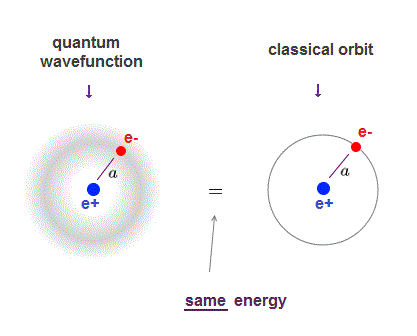Schrödinger equation of quantum mechanics gives exactly the same energy levels and electron's orbital radius as classical orbit in hydrogen.

Quantum mechanics also uses de Broglie wavelength, which determines the average electron's orbital radius.

The average electron's orbital radius in ground state hydrogen agrees with Bohr radius (= a ) in both quantum mechanics and classical orbit.

So even the lowest-energy electron keeps some distance (= a ) from the nucleus, as far as the orbit is an integer times de Broglie wavelength (= boundary condition in quantum mechanics ).

## Why quantum mechanics is useless ?

### [ Electrons cannot avoid each other in quantum mechanics. ]

(Fig.2)  ↓ Unreal quantum wavefunction = an electron everywhere !?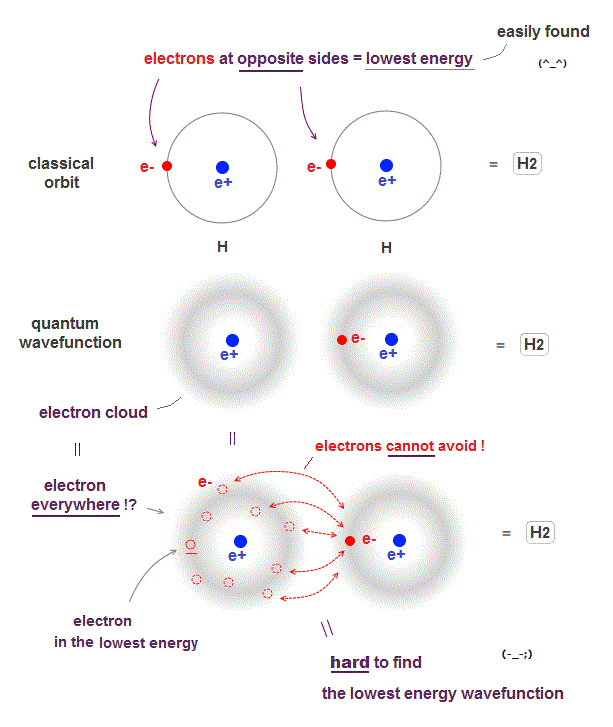Due to de Broglie wavelenth, an electron keeps some distance from the nucleus in both quantum wavefunction and classical orbit even in the ground state.

When two hydrogen atoms form hydrogen molecule, the lowest energy state is when two electrons just avoid each other as shown in Fig.2 upper.

In classical orbit, we can easily find this lowest energy state in any molecules.

For example, In methane (= CH4 ), the tetrahedral distribution of carbon's four valence electrons is the lowest energy (= electrons avoid each other ), which can be easily found.

The problem is quantum mechanical wavefunction gives vague electron cloud spreading over all space, which means each electron is always everywhere.

If an electron is vague electron cloud, electrons cannot avoid each other in quantum mechanics !

When an electron of one atom approaches another nuclei and another electron moves away to the opposite side, it is the lowest energy state (= Fig.2 upper ).

But quantum wavefunction must always spreads over all space (= electron's probability is Not zero in any points ), which always includes higher-energy electron's positions.

It means classical orbits can easily find the lowest-energy electrons' positions (= useful !), while quantum mechanics must take infinite time to find the lowest-energy fake solution from infinite choices (= useless, impractical ).

See this p.12,  this p.19

## Basic science is useless.

### [ Quantum mechanics cannot do even simple calculation ! ]

(Fig.2a)  ↓ Calculating two charges is impossible in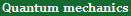?The current basic science is impractical, biologists and chemists ignore quantum mechanics due to useless Schrödinger equation ( this p.1 left ).

In classical physics, calculating force between two charges is very easy, but quantum mechanics can hardly calculate even this simple force !

Quantum mechanics treats each electron as vague cloud spreading over all space, which makes calculating electrons' force impractical in quantum mechanics.

If a single electron is spreading over space like quantum mechanics, electrons cannot avoid each other, which is unreal.

We have to calculate almost infinite patterns of Coulomb energies between small charges inside two electrons' clouds, and sum them up, which takes enormous time ( this p.4,  this p.8 ).

On the other hand, in classical electron = point charge, we just do only one calculation of Coulomb force between two electrons, which is much easier, and takes almost no time.

Why must a quantum mechanical electron always spread in all space ?  Because Schrödinger equation cannot distinguish between electron particle and de Broglie wave (= field ).

In quantum mechanics, de Broglie relation is expressed as derivative, which becomes infinity when an electron is a point charge = delta function.

But actual electrons are moving particles avoiding each other !
Effective nuclear charge which a helium electron feels is 1.69, bigger than 1, which means two electrons in helium are always avoiding each other ( this p.3, this last ).

And spreading electron clouds in quantum mechanics make it impossible to conserve total energy in multi-electron atoms, so wrong.

## Schrödinger's electron cannot be localized.

### [ Quantum mechanics must rely on "cheating", violating energy conservation law, so wrong. ]

(Fig.2b)  ↓ Electron's kinetic energy = derivative of wavefunction.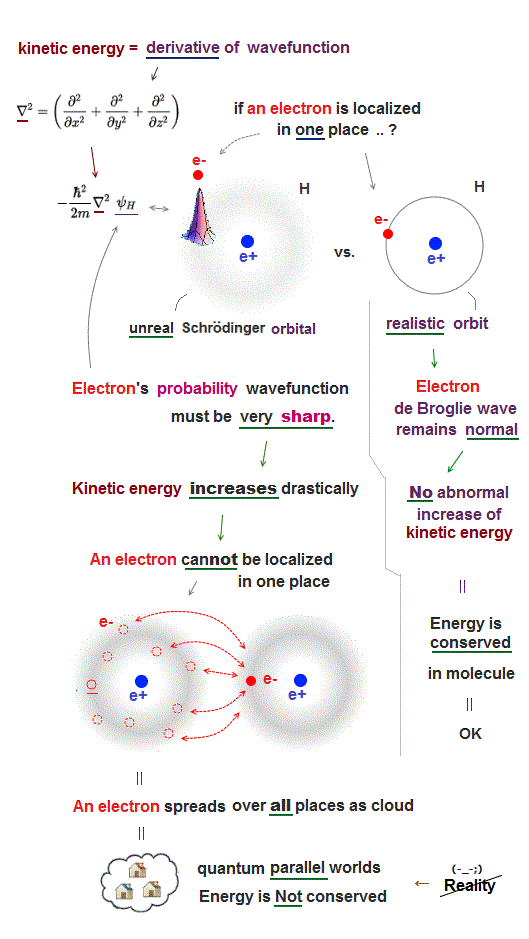The most crucial difference between quantum mechanics and realistic Bohr model is the definition of a particle and wave.

Quantum mechanics dismissed real entities such as an electron particle and de Broglie wave, instead, it just mixes a partcle and wave as one nonphysical wave function.

Therefore, in quantum mechanics, even a single electron spreads out over all space as fuzzy electron cloud (= whether an electron is a particle or wave is uncertain ).

They claim this fuzzy cloud densities at different areas show "different probabilities" of finding an electron.  ← This forced interpretation has No factual grounding.

If quantum mechanical picture is correct, a single particle has to pass through two slits at once like a ghost using fantasy parallel worlds to cause observed interference.

In Bohr's realistic picture, an electron just passes through one of two slits, and de Broglie wave around the electron can pass both slits, which model can naturally explain two-slit interference without relying on fictional quantum parallel worlds.

These quantum electron clouds cannot avoid other electrons, so to get molecular binding energy, quantum mechanics has to rely on "cheating" to lower total energy in unfair way which lowers only kinetic energy by violating energy conservation law and Virial theorem ( this p.3 ).

So quantum mechanical molecular bonds based on cheating have nothing to do with actual molecules, hence physically meaningless.  ← Another proof that quantum mechanics is wrong.

Quantum mechanical wavefunctions interpreted as "electron's probability" cannot avoid each other, because the electron cloud wavefunction must be always symmetrically distributed around the nucleus (= hence, the electron's probability also must be evenly distributed around the nucleus ).

If we try to localize each electron to one side of a nucleus to avoid another electron, quantum "electron-probability" wavefunction needs to be sharper at the electron's point, therefore drastically increasing the electron's kinetic energy which is expressed as a derivative of wavefunction.

For example, if we try to express quantum electron as a localized point particle like classical atomic model, the electron's probability wavefunction needs to be sharp delta function at the electron's position where the electron's kinetic energy (= derivative of wavefunction or delta function ) increases to infinity, which cannot form stable molecular bond (= lowering total energy is impossible ).

So the only realistic molecular model obeying energy conservation law by electrons avoiding each other must be like Bohr model where each electron is an actually moving particle and its de Broglie wave is generated around the electron particle (= so separating a particle from its wave is necessary. )

In this realistic Bohr model where an electron particle is separated from de Broglie wave generated around an electron particle, de Broglie wave has nothing to do with the electron's probability wavefunction, hence, an electron particle can be localized in one particular place without sharpening electron's probability wavefunction (= sharper wavefunction means shorter de Broglie wavelength and increasing electron's kinetic energy in unphysical quantum mechanics ) or increasing kinetic energy.

Mixing a electron particle and wave as "one weird probability wavefunction" as seen in quantum mechanical Schrödinger equation is unable to describe actual stable molecules.

## Hydrogen (= H2 ) molecule bond energy.

### [ Quantum mechanical electron cloud cannot generate Coulomb molecular bond,  so false ]

(Fig.2c)  ↓ Only real moving electrons can get enough attraction.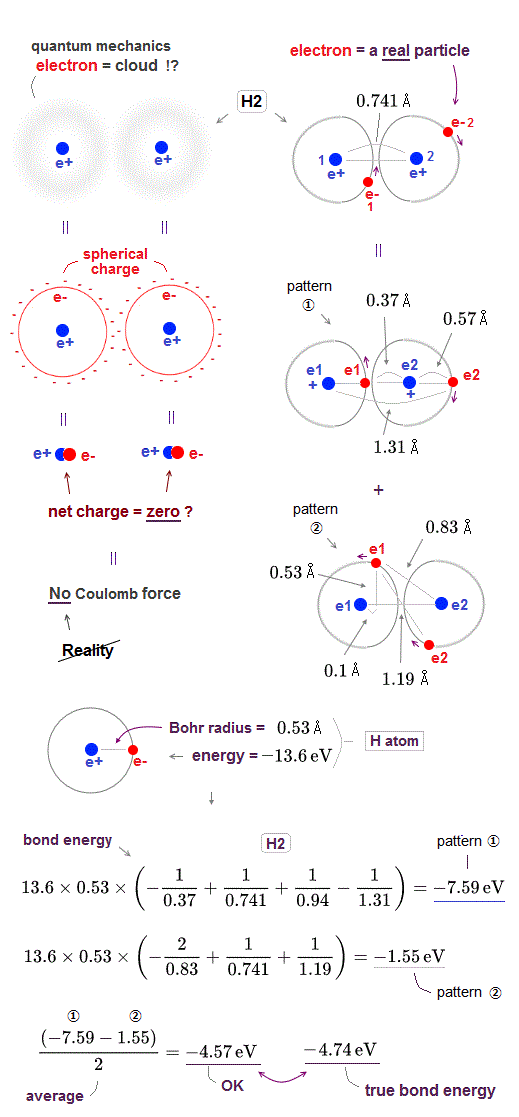The hydrogen atomic wavefunction of quantum mechanics is expressed as unphysical "electron cloud" where even a single electron must always spread over all space symmetrically around each nucleus.

This unrealistic quantum mechanical electron cloud can neither approach the other positive nucleus nor avoid the other negative electron, so it cannot generate enough "Coulomb attraction" to form molecular bond.

This electron cloud mixing up an electron particle and wave picture as one nonphysical wavefunction is caused by the fact that unrealistic quantum mechanics cannot distinguish a real electron from de Broglie wave in the "medium".

Electron cloud distributed "symmetrically" and evenly around each nucleus is equal to " spherical charge" (= so the center of this quantum electron cloud is just at the same position as a nucleus, overlapping it ) around a nucleus.  ← The net charge of electron cloud and nucleus (= overlapping at the same position ) is almost neutral = zero.  ← No Coulomb force !

Therefore, quantum mechanical electron cloud cannot generate Coulomb energy enough to bind H2 molecule, and it has to rely on "cheating" where total energy is Not conserved and kinetic energy is lowered illegitimately (= instead of lowering Coulomb potential energy legitimately ) using unreal indistinguishable electrons and nonphysical "exchange energy".

In this quantum mechanical "indistinguishable" electrons, each electron must always exist in all different atoms unrealistically, so quantum mechanical molecule cannot be used as practical basic structual tool.

On the other hand, in the realistic atomic model, each electron is "actually moving" (= able to approach the other positive nucleus and avoid the other negative electron ) to generate Coulomb attractive force enough to form molecular bond.

Here, we roughly estimate how much hydrogen (= H2 ) molecular bond ( Coulomb ) energy is generated by this realistic moving electron model.

The total energy and average electron' orbital radius of hydrogen atom are -13.6 eV and 0.53 Å (= 1 Å = 10-10 m ), respectively.  ← We use these values for calculation.

In hydrogen (= H2 ) molecule, the bond length between two hydrogen atoms is 0.741 Å and bond energy is -4.74 eV.

In pattern ①, the electron-1 approaches the nucleus-2 (= distance between the electron-1 and nucleus-2 is the shortest = 0.37 Å ), and the electron-2 avoids the electron-1 to the opposite side of the nucleus-2 (= distance between the electron-2 and nucleus-2 is elongated to 0.57 Å due to repulsive electron-1 ).

The Coulomb potential energy (= total bond energy including kinetic energy using Virial theory ) of this pattern ① between two H atoms 1 and 2 becomes -7.59 eV, which is the sum of Coulomb energy between electron-1 and nucleus-2 (= distance 0.37 Å), two nuclei (= distance 0.741 Å ), two electrons (= distance 0.94 Å ) and the electron-2 and nucleus-1 (= distance 1.31 Å ).

The Coulomb potential energy (= total bond energy ) of this pattern ② between two H atoms 1 and 2 becomes -1.55 eV, which is the sum of Coulomb energy between electron-1 and nucleus-2 (= distance 0.83 Å), two nuclei (= distance 0.741 Å ), two electrons (= distance 1.19 Å ) and the electron-2 and nucleus-1 (= distance 0.83 Å ).

So the average total bond energy of this realistic H2 molecule model becomes -4.57 eV which is good result close to experimental value -4.74 eV.

In H2 molecule, each electron' orbital radius shrinks more, because the number of the central positive nuclei increases to "two" from "one" of hydrogen atom, so considering shorter orbital radius can get this calculation value closer to true H2 molecular bond energy.

We need to consider other factors such as de Broglie wave interference where two orbits of H2 molecule have to be perpendicular to each other at their crossing point to avoid destructive interference which is realistic version of Pauli exclusion principle.

As shown in the pattern ① in the upper figure, the negative electron (= moving particle ) approaching the other positive nucleus and avoiding the other negative electron is the "main generator" of actual molecular bond energy.

So, the unrealistic quantum mechanical electron cloud which cannot approach the other nucleus or avoid the other electron cannot generate enough Coulomb attraction.  ← It has to rely on illegitimate cheating.  ← Quantum mechanics is false.

From the two basic conditions of Coulomb force (= of course, total energy must be cosnerved ) and electron's orbital length which must be an integer times de de Broglie wavelength to avoid destructive interference, we can know electron's "orbital radius" from which information, we can estimate Coulomb force between atoms and predict the direction in which chemical reaction will proceed.

But in unrealistic quantum mechanical molecule which has No such thing as real "forces", they can neither calculate real forces nor predict how each atomic chemical reaction is going, because they cannot distinguish different atoms or electrons.  ← Unbelievable.

In pattern pattern ① of real H2 molecule in the upper figure, the distance between electron-1 (= e1 ) and nucleus-1 is also shorter (= 0.37 Å ) than the original Bohr radius (= 0.53 Å ) of independent hydrogen atom.

Because when the electron-1 approaches the other nucleus-2, the electron-1 is attracted to this nucleus-2 in the opposite direction of nucleus-1.

So the electron-1 needs to be closer to the original nucleus-1 to increase Coulomb attraction between electron-1 and nucleus-1 and overcome the attraction from the other nucleus-2, staying in the orbit around the original nucleus-1.

As a result, the distance between electron-1 and nucleuc-1 becomes shorter in H2 molecule than the original Bohr radius of independent hydrogen atom, and this "shrinking orbital radius" when each electron passes between two positive nuclei (= this electron is attracted in the opposite directions by two positve nuclei ) is one of the main generators of lowering Coulomb potential energy to form real molecular bond.

## Why classical orbit is useful ?

### [ Classical orbits easily compute "force" besides energy. ]

(Fig.3)  ↓ Classical orbit vs. useless quantum molecule = No force.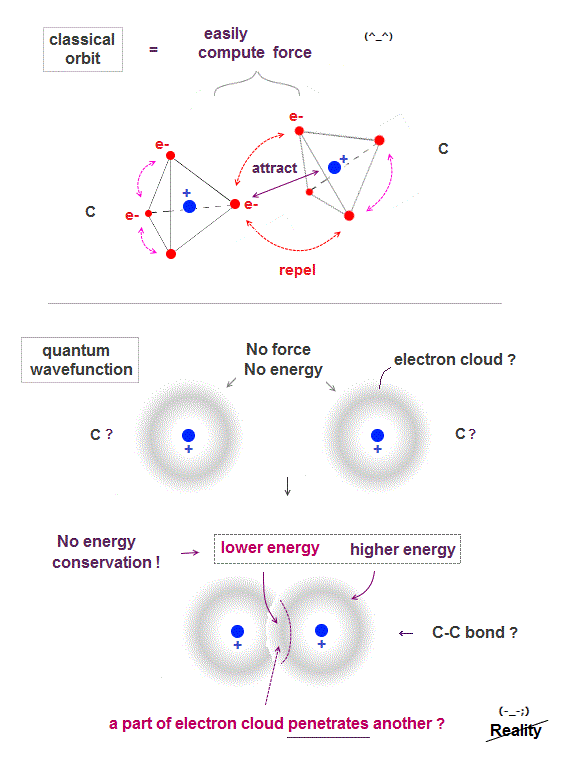In classical orbit, electrons are point particles separated from each other, so it can easily find the most stable (= the lowest energy ) electrons' position.

The lowest-energy state is when electrons just avoid each other between and inside atoms.

After finding the lowest-energy state, we can easily calculate force between atoms, and predict which direction each atom will move in new chemical reaction.

Quantum mechanical wavefunction is vague electron cloud, which cannot avoid each other, so it's very hard (= impossible ! ) to find the lowest-energy molecular wavefunction.

If a single electron is Not a point particle but spreading over all space as cloud, two electrons don't feel repulsion from each other, when they are outside of each other, as seen in spherical charge.

Only when two wavefunctions penetrate each other (= inside spherical electron charge ), it feels more attraction from another nucleus, causing molecular bond ?

Quantum mechanics needs infinite time to find the lowest-energy wavefunction from infinite choices, artificially changing trial wavefunctions and the amount of electron penetration.

Quantum mechanics cannot calculate "force", which makes itself useless, because "energy" does not tell us "direction" in which atoms will move in chemical direction.

Estimating force is much easier, because we only consider a few electrons between atoms, while when computing energy, we have to consider the "whole orbital" (= all other many electrons involved ).

The worst point of quantum mechanics is its molecule cannot satisfy energy conservation ( an electron penetrating another electron is lower energy than outside ).

## Why Pauli principle is wrong ?

### [ Pauli principle violates energy conservation ! ]

(Fig.4)  ↓ Overlapped part is lower energy = No energy conservation !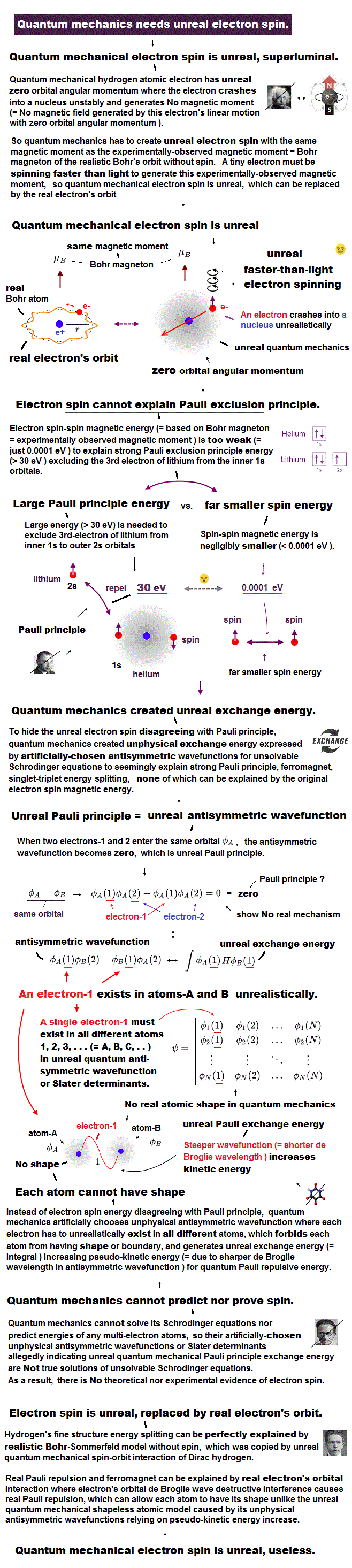The problem is Schrödinger's atoms cannot satisfy energy conservation law, so unreal.

Even when two hydrogen atoms overlap, each hydrogen's electron energy is conserved only with respect to each nucleus, Not to another nucleus.

A part of electron cloud penetrating another electron cloud feels more attraction , this overlapped wavefunction part (= feels both nuclei ) is lower energy than outside of cloud (= feels only one nucleus ), so total energy is Not conserved in different positions.

When wavefunction is antisymemtric (= when exchanging r1 and r2, it becomes minus ), it causes Pauli exclusion principle, they insist.

In Fig.4 lower (= antisymemtric, Pauli ), overlapped part (= lower energy ) is cancelled, leaving only higher energy part, so the total is higher energy, which causes Pauli repulsion force ?

This Pauli principle by quantum mechanics depends on unreal condition (= energy is Not conserved between overlapped and non-overlapped parts ), so untrue.

## Hydrogen molecules (H2) in quantum mechanics use non-existent exchange energies.

### [ Antisymmetric wavefunctions give fictitious exchange (= overlapped ) energies of fake molecular attraction or Pauli repulsion. ]

(Fig.5)  ↓ Artificial antisymmetric wavefunction = Pauli exchange energies ?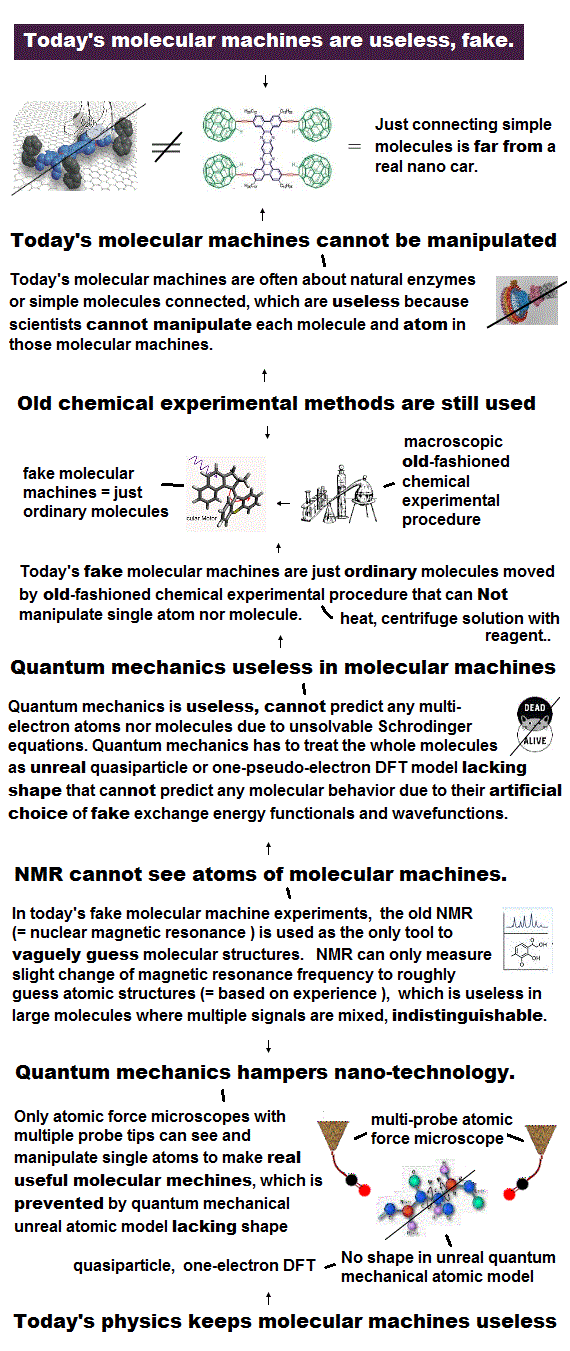Basically, quantum mechanical only calculation tool = Schrödinger equations cannot be solved for any multi-electron atoms or molecules except for the simplest hydrogen atom ( this p.21 ), so quantum mechanics is completely useless with No power to predict anything.

Of course, Schrödinger equation for a two-electron hydrogen molecule (= H2 ) is also unsolvable ( this p.4 ). = The true H2 molecule wavefunction is unknown forever.

Physicists have to artificially choose fake approximate solutions or trial wavefunctions with many freely-adjustable parameters using the methods called variational method, which cannot predict any atomic energies by solving the unsolvable Schrödinger equations.

They often choose the product of a hydrogen atom A (= H wavefunction φa ) and a hydrogen atom B (= H wavefunction φb) as an approximate (=fake) H2 molecule wavefunction.

Schrödinger energy equation (= called Hamiltonian H ) for hydrogen molecule consists of the kinetic energy terms expressed as derivatives of electron-1 and electron-2 wavefunctions, and Coulomb potential energy terms (= Coulomb attractions between electron-1 and two H nuclei-A and B, Coulomb attractions between electron-2 and two H nuclei-A and B, Coulomb repulsion between electron-1 and electron-2, repulsion between nucleus-A and nucleus-B ).

Of course, unsolvable Schrödinger equations for hydrogen molecule (= H2 ) cannot have the exact solution, hence, physicists choose the product of two hydrogen atomic 1s wavefunctions as the form of (anti-)symmetric wavefunctions obeying the quantum mechanical unphysical Pauli principle exchange rule, which are proved physically wrong, as I explain later.

When two electrons have the opposite up and down spins (= antisymmetric spins or singlet ), the H2 molecular (spacial) wavefunction is supposed to form the symmetric wavefunction (= ψ+  = hence, total wavefunction = spin + spacial parts should always take anti-symmetric form ) which allegedly causes fake molecular attractive exchange energies.

When two electrons have the same spins (= up-up or down-down spins = spin part is symmetric, triplet ), the H2 molecular wavefunction becomes antisymmetric (= ψ- ) which allegedly causes fictitious Pauli repulsive exchange energies.

Physicists have to put the unsolvable H2 molecular Schrödinger equation (= H ) between chosen (anti-)symmetric H2 molecular wavefunctions (= ψ± ) and integrate them to obtain fake average total energies E ( this p.3 ) instead of solving the unsolvable multi-electron Schrödinger equations.

And this integration gives the unphysical exchange (= overlapped ) energy terms (= exchange integral ) which are supposed to determine whether the molecule forms the attractive bond or the repulsive anti-bond depending on whether the chosen (spacial) wavefunction is symmetric or antisymmetric wavefunction ( this p.3-4,  this p.15-16 ).

We can prove, this quantum mechanical Pauli exchange energies based on unphysical antisymmetric wavefunctions are intrinsically wrong, due to violation of total energy conservation law.

This quantum mechanical ad-hoc exchange energy or exchange integral often disagrees with experimental values.

For example, the experimental energy levels of helium 1s2p 3P (= triplet ) and 1s2p 1P (= singlet ) are higher than 1s2s and lower than 1s3s.

But the energy values obtained by this ad-hoc integration of helium Schrödinger equations and antisymmetric wavefunctions ( this p.6 ) are different and wrong = 1s2p 1P (= -50.3 eV ) is higher than 1s3s (= -55.25 eV ),1s6s (= -55.43 eV ), and 1s2p 3P (= -59.0 eV ) is lower than both 1s2s (= -57.67 eV ).  ← There is No experimental verification of artificial Pauli exchange energies.

## Proof that quantum mechanical wavefunctions are false and impossible.

### [ Conservation of the total energies is violated in all quantum mechanical antisymmetric wavefunctions. ]

(Fig.6)  ↓ If H2 molecular symmetric (= ψ+ ) and antisymmetric (= ψ- ) wavefunctions are the solutions of Schrödinger equations .. ?  → Total energy is Not conserved.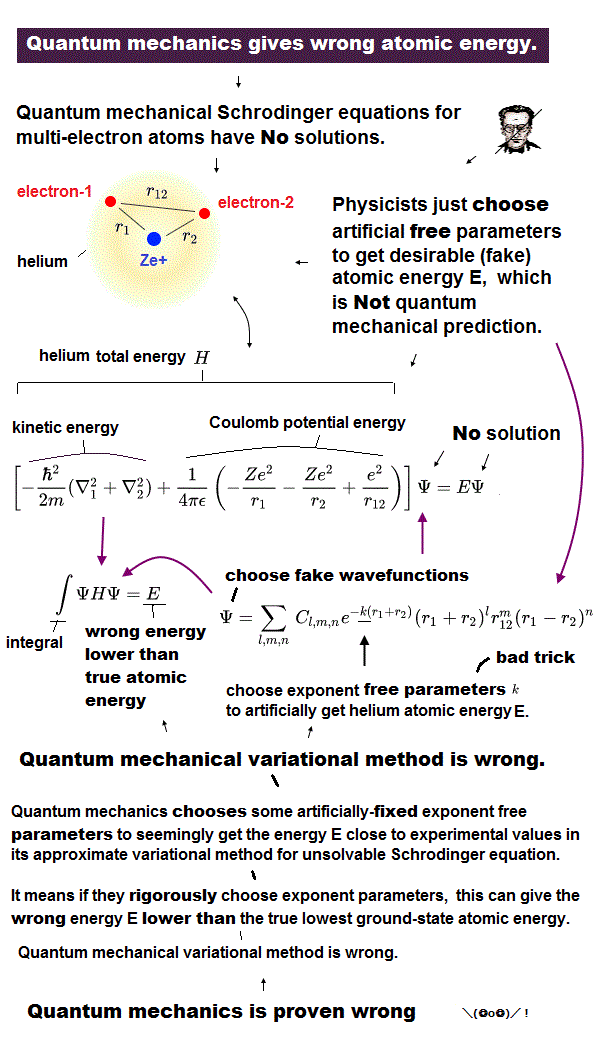The sum of electrons' kinetic energies and Coulomb electric potential enregies is the total energy E which must be always conserved and constant ( this p.1-last ).

Actually, the only exact wavefunction or solution of one-electron hydrogen atom can be obtained by solving its Schrödinger equation under the constant and conserved total energy E ( this p.2,  this p.2-1st-paragraph ).

So the conservation of the total energy E in any electrons' positions is the indispensable condition for solving Schrödinger equations and getting the exact wavefunctions (= exact solutions ).

Here we can rigorously prove that the quantum mechanical (anti-)symmetric wavefunctions needed for Pauli principle can neither conserve the constant total energy E nor give the exact wavefunctions.

So quantum mechanical antisymmetric wavefunctions can never be the exact solutions of Schrödinger equations, no matter what approximate trial wavefunctions are chosen.  → quantum mechanics is false.

Quantum mechanics states that all wavefunctions must take (anti)symmetric forms to satisfy Pauli exclusion principle.

H2 molecule wavefunction is also required to take the symmetric form (= ψ+ = φa(r1)φb(r2) + φb(r1)φa(r2),  this p.3-middle,  = when two spins are up and down = antisymmetric or singlet ) or the anti-symmetric form (= ψ- = φa(r1)φb(r2) - φb(r1)φa(r2),  = when two spins are the same symmetric or triplet ).

So H2 molecular wavefunction must take either of the two forms below.

ψ+ = φa(r1)φb(r2) + φb(r1)φa(r2)  ← symmetric or
ψ- = φa(r1)φb(r2) - φb(r1)φa(r2)  ← antisymmetric

φa(r1) is hydrogen atom-Ha wavefunction φa of electron-1
φa(r2) is hydrogen atom-Ha wavefunction φa of electron-2
φb(r1) is hydrogen atom-Hb wavefunction φb of electron-1
φb(r2) is hydrogen atom-Hb wavefunction φb of electron-2

↑ Both electrons 1 and 2 must unrealistically exist in two separate hydrogen Ha and Hb atoms at the same time using parallel worlds.

We insert these (anti-)symmetric wavefunctions into the ordinary Schrödinger equations where the total energy E is the sum of kinetic energy + Coulomb potential energy, and confirm this Schrödinger equation can Not be solved under the constant conserved total energy E, unlike the only solvable hydrogen atomic Schrödinger equation.

Coulomb potential energy terms are basically symmetric with respect to an electron-1 and electron-2 (= even if we exchange labels of the electron-1's coordinate r1 and electron-2's coordinate r2, r1 ↔ r2, the Coulomb potential energy equation does not change ).

On the other hand, the kinetic energy terms expressed as derivatives of wavefunctions become asymmetric with respect to the electron-1 (= r1 ) and electron-2 (= r2 ).

Because the hydrogen atom-A's wavefunction (= φa ) and the hydrogen atom-B's wavefunction (= φb ) are completely different things with different nuclear centers at different positions.

↑ Kinetic energy operators (= derivative ) acting on these two different hydrogen atoms φa and φb give two different asymmetric kinetic energy functions of two electrons' coordinates r1 and r2, unlike the always-symmetric Coulomb energy functions with respect to two electrons' coordinates r1 and r2.

Hence those electrons' kinetic energies expressed as derivatives of the different hydrogen atomic wavefunctions also become completely different, irrelevant and asymmetric.

This poses a serious problem, when we try to conserve total energy E using quantum mechanical wavefunctions.

Because in order to solve the exact Schrödinger equation, the electrons' kinetic energy terms must just cancel the Coulomb potential energy terms to become the constant total energy E in any electrons' positions.

↑ But the asymmetric kinetic energy terms ( with respect to electrons-1 and 2 variables r1 and r2 ) cannot cancel the symmetric Coulomb energy terms.

Hence, the conservation of the total energy E or solving Schrödinger equation by kinetic energies canceling out Coulomb potential energies is impossible in any quantum mechanical multi-electron atoms and molecules obeying nonphysical Pauli antisymmetric wavefunction rule.

## Quantum mechanical wavefunctions can never conserve total energy, so quantum mechanics is wrong.

### [ Quantum mechanical Pauli antisymmetric wavefunctions can never be true solutions conserving the constant total energy E. ]

(Fig.7)  ↓ If quantum mechanical (anti)symmetric wavefunctions (= ψ± ) are solutions, their Schrödinger equations are unsolvable, unable to conserve the constant total energy E.Continued from Fig.6, Schrödinger equations for H2 hydrogen molecular (anti-)symmetric wavefunctions consisting of hydrogen atom-Ha wavefunction (= φa ) and hydrogen atom-Hb wavefunction (= φb ) can be equal to the last form of Fig.7.

In order for the total energy E to be constant and conserved in quantum mechanical atoms and molecules expressed as antisymmetric wavefunctions, the last identity ( Schrödinger ) equation of Fig.7 must be satisfied where the exchange of the electron-1 (= r1 ) and electron-2 (= r2 ) variables or coordinates on the left side must give the right side of the equation ( except for the sign ± added ).  ← This is impossible.

Because hydrogen atom-Ha wavefunction (= φa ) and hydrogen atom-Hb wavefunction (= φb ) are completely different things with different nuclear centers' positions.  ← The left side and right side cannot be equal in the last identity equation of in Fig.7

So this identity equation can never be satisfied unless hydrogen atom-Ha wavefunction (= φa ) and hydrogen atom-Hb wavefunction (= φb ) are completely the same wavefunction with the same nucleus existing at the same position.  ← This is Not a molecule, and it's impossible for two positive Ha and Hb atomic nuclei to overlap each other ( φa = φb ) in the same position.

↑ In this impossible case of two H atoms existing at the same position (φa = φb ), the total energy of symmetric (= E+ ) and antisymmetric (= E- ) wavefunction just become the same ( E+ = E- ) = symmetric and antisymmetric wavefunctions make No difference = quantum mechanical spin's directions, antisymemtric wavefunctions and exchange energies become meaningless.

As a result, if quantum mechanical antisymmetric wavefunctions (= for satisfying Pauli principle ) are solutions of atomic or molecular Schrödinger equations, the total energy E can never be constant or conserved.

It means quantum mechanics itself is wrong.

As I said in Fig.6, these asymmetric (= with respect to electrons-1 and 2 ) kinetic energy terms cannot cancel symmetric Coulomb energy terms.  ← The constant conserved total energy E is impossible in quantum mechanical molecules satisfying Pauli principle's antisymmetric wavefunction rule.

## Pauli principle by quantum mechanics is wrong.

### [ If total energy E is conserved, both symmetric and antisymmetric wavefunctions give the same energy. → No Pauli exclusion principle ! ]

(Fig.8)  ↓ Energy is conserved = E± = E.  ← No Pauli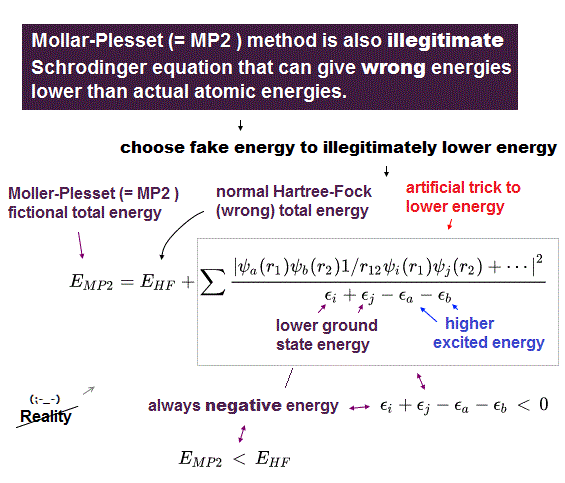When Schrödinger equation has the right solution (= if Schrödinger equation is solvable like an one-electron hydrogen atom ), the total energy E, which is equal to the sum of electrons' kinetic energies and Coulomb potential energies, must be always conserved and constant in any electron's position (= r ) within the solution wavefunction.

Because the solvable Schrödinger equation becomes an identical equation with respect to any electrons' variables or coordinates (= electron-1 position = r1, and electron-2 position = r2 ) under the constant total energy E where the total energy E is conserved and constant inside a molecule.

Conservation of the total energy E is the necessary condition for Schrödinger equations to have the exact solution. So if we can prove the total energy E of quantum mechanical multi-electron atoms and molecules can Not be conserved or constant, quantum mechanics itself is proved wrong.  ← This will be shown here.

Quantum mechanics demands that any multi-electron atoms and molecules take nonphysical anti-symmetric wavefunctions to satisfy Pauli exclusion principle where the sign of the entire wavefunctions becomes the opposite when exchanging any two electrons' variables or coordinates (= r1 ↔ r2 ).

Pauli exclusion principle states that only two electrons with the opposite different spins (= up and down ) can occupy the same state or orbital, which is why lithium's 3rd electron cannot enter the inner 1s orbitals which already have two electrons with the opposite spins.

If two electrons with the same spins (= up-up or down-down spins ) enter the same orbital, it means the antisymmetric wavefunction (= φa(r1)φb(r2) - φb(r1)φa(r2) ) includes two same electrons' wavefunction in both orbital and spin, and resultantly becomes zero ( this p.2,   when φa = φb,  φa(r1)φb(r2) - φb(r1)φa(r2) becomes φa(r1)φa(r2) - φa(r1)φa(r2) = zero ).  ← Pauli principle ?

↑ Quantum mechanics clarifies No more detailed mechanism of Pauli principle, so the realistic Pauli principle mechanism is still unknown.

When two electrons have the opposite up and down spins called singlet (= spin part is antisymmetric ), the spacial wavefunction must be symmetric for the entire wavefunction, which is the product of spacial and spin-part wavefunctions, to be antisymmetric ( this p.11 ).

When two hydrogen atoms Ha and Hb make attractive H2 molecular bond, this H2 molecular (spacial) wavefunction must be symmetric ( this p.3-middle ), because two electrons of two Ha and Hb atoms have the opposite (= antiparallel or singlet ) spins.

When the 3rd electron of the 3rd hydrogen Hc atom tries to enter this H2 molecular bond which already contains two electrons of Ha and Hb hydrogen atoms with opposite spins, this 3rd electron allegedly feels strong Pauli repulsion and cannot from three H3 molecular bond (= Ha-Hb-Hc ), quantum mechanics claims.  ← But actually, quantum mechanical Pauli repulsion is too weak to stop unreal H3 molecular formation.

This 3rd electron of Hc atom have the same up-up or down-down spins (= called parallel or triplet spins ) with either of the two Ha or Hb atomic electrons already occupying H2 molecular bond's orbital.

So this 3rd electron must form antisymmetric (spacial) wavefunction causing Pauli repulsive exchange energies to stop unreal H3 molecule from appearing ( this 3rd paragraph ).

This means the total energy E+ of symmetric (spacial) wavefunction (= φa(r1)φb(r2) + φb(r1)φa(r2)  = antiparallel singlet spin ) generating attractive molecular bonds must be lower than (= Not same as ) the total energy E- of antisymmetric wavefunction (= φa(r1)φb(r2) - φb(r1)φa(r2)  = antibonding with parallel triplet spins ) allegedly causing Pauli repulsion ( this p.3,  this p.8-9 ).

In fact, if the total energy E± could be constant and conserved (= if quantum mechanical antisymmetric wavefunctions could be the exact solutions of Schrödinger equations conserving the total energies ), the energy difference between symmetric (= which should generate lower energies enough to form attractive molecular bonds ) and antisymmetric (= which should generate higher energies enough to cause Pauli repulsion ) wavefunctions would disappear ( E+ = E- ) !

It means if the total energies are conserved and constant, quantum mechanical molecules can cause neither molecular attractive nor Pauli repulsive energies, hence, quantum mechanics is false.

If the total energy (= E± ) is conserved and constant, quantum mechanical (anti-)symmetric wavefunctions must satisfy the ordinary Schrödinger's energy identical equations Hψ± = E±ψ± where H is the energy equation called Hamiltonian operator like the solvable one-electron hydrogen atomic Schrödinger equation.

For the total energy E± to be constant and conserved, electrons' kinetic energy terms must cancel out Coulomb potential energy terms in any electrons' positions.

But as I said, the kinetic energy terms of two electrons belonging to two different hydrogen Ha and Hb molecules with two different center nuclei (= asymmetric with respect to electrons-1 and -2 coordinates ) cannot cancel the Coulomb potential energy terms (= symmetric with respect to electrons-1 and -2 coordinates, as shown in this upper H ).

So in order for the kinetic energies to cancel Coulomb energies for getting the constant total energy (= by satisfying the condition of energy H - E± = 0, where Hamiltonian H consists of (asymmetric) kinetic and (symmetric) Coulomb potential energy terms ), two hydrogen atoms Ha and Hb wavefunctions must be exactly the same one sharing the same nucleus existing in the same position to make their kinetic energy terms symmetric and cancel symmetric Coulomb potential energy terms, which is No longer a H2 molecule consisting of two H atoms apart from each other.

And in this case of conserving total energy E± in quantum mechanical antisymmetric wavefunctions ( Hψ± = E±ψ± ), the molecular attractive energy (= E+ ) and Pauli repulsive energy (= E- ) become unrealistically the same ( E+ = E- ) due to the same Ha and Hb wavefunctions with the same nucleus (= φa = φb ).

It contradicts the fact that there is some energy difference between attractive molecular bonding symmetric (= with anti-parallel or singlet spins ) and Pauli repulsive anti-bonding antisymmetric (= with parallel or triplet spins ) wavefunctions.  ← Quantum mechanical Pauli antisymmetric wavefunction rule disagrees with the energy conservation law !

If thre is no energy difference between symmetric and antisymmetric wavefunctions (= E+ = E- = E ), the energy level's splitting between atomic singlet and triplet states would be gone, disagreeing with the experimental results.

As a result, quantum mechanical Pauli antisymmetric wavefunctions can neither conserve the total energy E nor become the true solutions of Schrödinger's multi-electron equations, hence, quantum mechanics is proved to be false.

This is why nonphysical exchange energies must be expressed only as "exchange integral" instead of solving Schrödinger equations ( this p.3 ).

↑ In order to obtain the total energy E, the only solvable one-electron hydrogen atomic Schrödinger equation does Not need to integrate the chosen fake trial wavefunctions unlike unsolvable helium and other molecules.

## What force causes Pauli principle ?

### [ Quantum mechanics is wrong in Pauli exclusion principle. ]

(Fig.9)  ↓ Total energy is Not conserved in different area → Pauli principle ?We explain why quantum mechanical Pauli principle is wrong.

Pauli exclusion principle says two electrons cannot occupy the same orbital, which is why the 3rd electron of lithium cannot enter 1s orbital.

The force of this Pauli exclusion is mystery, as strong as Coulomb force, but Not fundamental force ?

Electron spin lacks reality, its spinning speed is superluminal.  Electrons spin is too weak to cause Pauli exclusion force.

They say this Pauli exclusion force is caused by "antisymmetric wavefunction", where the sign of total wavefunction becomes the opposite, when exchanging variables of two functions.

So when two electrons are in the same orbital, the total wavefunction becomes zero, which nonphysical concept really causes Pauli principle ?

This Pauli principle by quantum mechanics is just wrong.

The square of wavefunction (= electron probability ) must be always 1, so the total wavefunction cannot be zero (= quantum Pauli is invalid ), because we have to get it back to 1 by multiplying it by the normalization constant.

In fact, quantum Pauli principle needs violation of energy conservation !

They use H2 molecule consisting of two H atoms.  In this case, there is energy difference between symmetric and antisymmetric wavefunctions, which violates energy conservation.

Only inside each H atom, the total energy is conserved independently from another electron, because they use H atom wavefunction, which can be solved.

It means the total energy of the whole H2 molecule is Not conserved, where the overlapped part (= close to both nuclei ) is in lower energy than nonoverlapped part.

So antisymmetric wavefunction which cancels overlapped part gives higher total energy, which Pauli exclusion force is caused by violation of energy conservation, so unreal.

If the total energy of molecule is conserved, both overlapped and non-overlapped parts must give the same total energy, invalidating quantum mechanical Pauli principle.

Just exchanging variables (= 1 and 2 ) in molecule doesn't change its total energy, so there must Not be energy difference between symmetric and antisymmetric wavefunctions, if they are exact solutions (= conserve total energy ).

Schrödinger equation has No exact solution except H atom.  They just choose fake solution from infinite choices (= infinite time is needed ! ) and integrate it instead of solving it, which cannot predict anything.

In 1920s without computer, physicists couldn't compute complicated integral.  All they could do was express molecule mixing simple H atom orbitals.

In pi bond, H atomic s and p orbital is canceled ?  1s and 2s H atom wavefunctions cancel each other, invalidating antisymmetric Pauli principle ( this p.2 ) ?  These artificial models rely on empirical parameters.

All these rough approximations violate total energy conservation of molecules, they use this violation as quantum Pauli principle, which is unreal.

Experimental proof of de Broglie wave interference and radiation pressure indicates some "medium pressure" causes real Pauli exclusion force.

## Quantum molecule is "cheating".

### [ Total energy is Not conserved in quantum mechanics. ]

(Fig.10)  ↓ Total energy is Not conserved in H2 molecule. → bond energy ?An electron of Schrödinger equation must always spread over all space as electron cloud, so electrons cannot avoid each other in quantum mechanical molecules.

It means quantum mechanical molecules always give higher energy than real molecules (= electrons of real molecules can avoid other electrons ).  Then, how do those quantum molecules form stable bonds between atoms ?

In fact, quantum mechanics relies on "cheating" in molecular bonds.
They say H2 molecule approximately consists of two H atomic 1s wavefunctions.

In each H atom, the total energy (= kinetic + Coulomb potential energy ) is constant, conserved in any electron's positions.

When an electron approaches H atom nucleus, Coulomb potential energy is lower, its kinetic energy becomes higher, therefore the total energy is unchanged = conserved constant.

But when they use this H atom wavefunction as a part of H2 molecule, the total energy is Not conserved.  ← quantum mechanical molecule is wrong.

Because even when an electron approaches another H atom nucleus (= Coulomb potential energy decreases ) in H2 molecule, its kinetic energy still keeps decreasing, so the total energy keeps decreasing, violating energy conservation law.

The total energy of each electron is seemingly conserved only around the area near either of two nuclei closer to the electron.

But each hydrogen wavefunction spreading over all space can reach another distant nucleus, decreasing both its kinetic energy and Coulomb potential energy.

As a result, the total energy = kinetic energy + Coulomb potential energies is Not conserved or constant in quantum mechanical molecule.

Quantum mechanical molecule uses this violation of energy conservation (= decreasing kinetic energy in an unfair way ) as fake binding energy, which is nonsense and unreal.

Each H atomic 1s wavefunction includes only one crest, where steep slope means higher kinetic energy, gentle slope is lower kinetic energy.

For the total energy to be conserved also in H2 molecule, the basic wavefunction must consists of at least two crests.

Quantum mechanics is "cheating" to form molecules, so false.

## Wrong math trick in quantum molecule.

### [ Quantum mechanics uses dirty trick to lower total energy. ]

(Fig.11)  ↓ Dirty trick in hydrogen molecules H2.Quantum mechanics says hydrogen molecule (= H2 ) approximately consists of two Hydrogen 1s wavefunctions.

Each Hydrogen 1s wavefunction belongs to either nucleus of H2 molecule in each term (= In the different term, two electrons are swapped, the total energy remains the same, so we think only about one term ).

For example, in the first term of ψ+ wavefuncton of Fig.11, the electron 1 (= r1 ) belongs only to Hydrogen-1 nucleus (= φa ).

Coulomb attractive potential energy of Hydrogen molecule includes ones between each electron and both of two Hydrogen nuclei-1 and 2.

Coulomb attractive potential energy of the electron-1 consists of two terms ( -e2/ra1 and -e2/rb1 ) from both two nuclei.

So as electron 1 is farther away from Hydrogen-1 nucleus, its Coulomb potential energy becomes higher (= -e2/ra1 is higher ) and its kinetic energy becomes lower to cancel the increasing potential energy to conserve total energy E.

But as as electron 1 is farther away from Hydrogen-1 nucleus, the electron is closer to Hydrogen-2 nucleus and its Coulomb potential energy becomes lower (= -e2/rb1 is lower ), but its kinetic energy remains lower, too.

This means violation of conservation of total energy.
Because as the electron's potential energy is lower, its kinetic energy also becomes lower.

This is a dirty, wrong math trick for quantum mechanics to lower the total energy of molecules in a wrong way.

So quantum mechanics is false, and its wavefunction lacks physical reality.

## H2 molecule energy calculation cheating.

### [ Overlap (= exchange ) integral exacerbates "cheating". ]

(Fig.12)  ↓ Overlap integral exacerbates violation of energy conservation.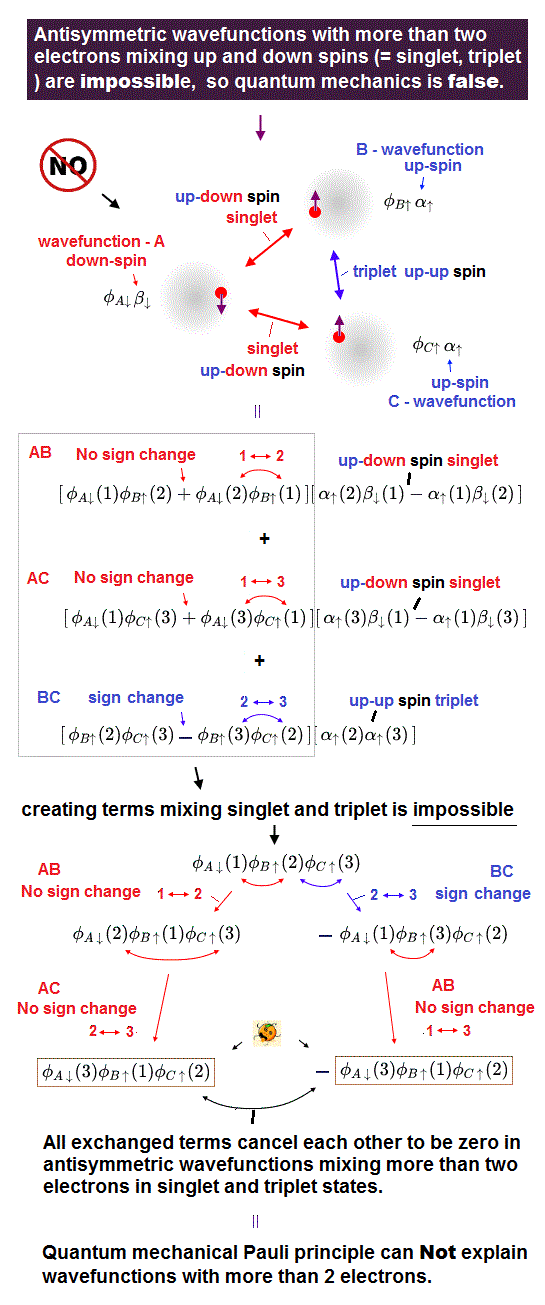In calculating Schrödinger equation of hydrogen (H2) molecule, we often pick up two hydrogen 1s atoms's wavefunctions (= φa and φb ) as approximate (= fake ) H2 molecule solution.

To seemingly get molecular bond energy by lowering total energy in quantum mechanical illegitimate "cheating", they use symmetric wavefunction where exchanging two electrons' variable numbers (= r1 and r2 ) gives the same original form of wavefunction (= this is just an artificial rule ).

An electron-1 (=r1) shares two hydrogen atomic wavefunctions φa(r1) and φb(r1).
An electron-2 (=r2) also shares two hyrogen atomic wavefunctions φa(r2) and φb(r2).  See this p.3

By integrating this fake H2 solution with Hamiltonian H (= total energy ), we obtain overlap (or exchange ) integral ( this p.14, this last ).

As I said, quantum mechanical molecule has to rely on "cheating" to lower total energy and get some molecular bond energy.

Kinetic energy of Hamiltonian H acts on hydrogen atom φa(r1) in Fig.12.  This electron-1 kinetic energy keeps decreasing even when the electron-1 approaches another H2 atomic nucleus and lowers potential energy.

Because this electron-1 is supposed to conserve total energy only around H1 atomic nucleus.  As the electron-1 gets farther away from H1 atomic nucleus (= approaching another H2 atomic nucleus ), its kinetic energy decreases offsetting higher potential energy between electron-1 and H1 atomic nucleus.

The energy change between electron-1 and another H2 atomic nucleus clearly violates total energy conservation, because both kinetic and potential energies decrease when the electron-1 approaches another H2 atomic nucleus.

Overlap (= exchange ) integral enhances this illegitimately lowered kinetic energy by multiplying φa by φb (= this amplitude is higher around H2 atomic nucleus ).

Lowering kinetic energy (= enhanced by overlap integral in symmetric wavefunction ) by violating total energy conservation and Virial theorem is the origin (= cheating ) of quantum mechanical molecular bonds.

This "cheating" shows quantum mechanical wavefunction is unable to form molecular bonds in the right and legitimate way, so quantum mechanics is false.2020/3/11 updated. Feel free to link to this site.